# Texas Go Math Grade 4 Unit 4 Answer Key Geometry and Measurement

Refer to our Texas Go Math Grade 4 Answer Key Pdf to score good marks in the exams. Test yourself by practicing the problems from Texas Go Math Grade 4 Unit 4 Answer Key Geometry and Measurement.

## Texas Go Math Grade 4 Unit 4 Answer Key Geometry and Measurement

Show What You Know

Check your understanding of important skills.

Identify Two-Dimensional Shapes Write the number of sides and vertices.

Question 1.___________ name
Triangle
3 vertices
3 sides
Explanation:
A triangle is a polygon with three sides having three vertices.
The angle formed inside the triangle is equal to 180 degrees.

Question 2.____________ name
Rhombus
4 vertices
4 sides
Explanation:
rhombus is a quadrilateral with four equal-length sides and opposite sides parallel to each other.
All rhombuses are parallelograms, but not all parallelograms are rhombuses.
All squares are rhombuses, but not all rhombuses are squares.

Classify Angles Classify the angle. Write acute, right, or obtuse.

Question 3.Right angle.
Explanation:
An angle which measures exactly 90° is called a right angle.
It is generally formed when two lines are perpendicular to each other.

Question 4.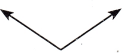Obtuse angle.
Explanation:
An angle that measures greater than 90° is known as the obtuse angle.

Question 5.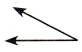Acute angle.
Explanation:
An angle which measures less than 90° is called an acute angle.

Time to the Half Hour Read the clock. Write the time.

Question 6.one and half an hour
Explanation:
Long hand shows minutes.
Short hand shows hours.
So, long hand is on 6 and minutes hand is between 1 and 2.
Thus the time in clock shows one and half an hour.

Question 7.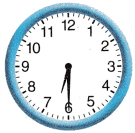Six and half an hour
Explanation:
Long hand shows minutes.
Short hand shows hours.
So, long hand is on 6 and minutes hand is between 6 and 7.
Thus the time in clock shows six and half an hour.

Question 8.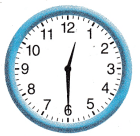Twelve and half an hour
Explanation:
Long hand shows minutes.
Short hand shows hours.
So, long hand is on 6 and minutes hand is between 12 and 1.
Thus the time in clock shows Twelve and half an hour.

Vocabulary Builder

Preview Words

acute angle
acute triangle
✓ cups
✓ decimeters
✓ gallons
✓ kilometer
line
✓ mile
✓ milliliter
✓ millimeter
obtuse angle
obtuse triangle
parallel lines
perpendicular lines
point
✓ pound
protractor
ray
rectangle
right triangle
✓ second
square
✓ tons

Sort words with a ✓ using the Venn diagram.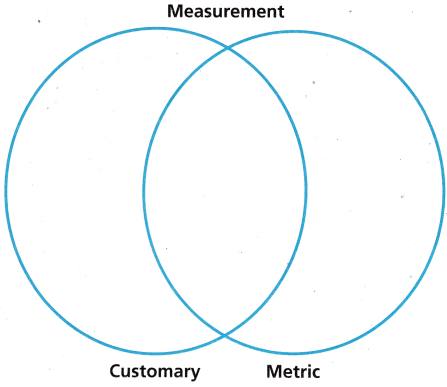Explanation:
The customary system of measurement, also called the U.S. Customary System, is based on the English system of measurement.
In math, the customary system can be defined as a set of weights and measures used for measuring length, weight, capacity and temperature.
The SI Units (standard International System of Units) gives proper definitions for meter, kilogram and the second.
It also specifies and defines four different additional units :
Kelvin- temperature Ampere – Electric Current Candela- luminous Intensity Mole – Material Quantity.

Complete the sentences by using the preview words.
Question 1.
An angle less than 90° is called an ____________ .
Acute angle.

Question 2.
A triangle with one 90° angle is called a ____________ .
Right angle

Question 3.
A quadrilateral with two pairs of parallel sides, four sides of equal length, and four right angles is called a ____________ .
Rectangle.

Question 4.
A pair of lines in the same plane which never cross and are always the same distance apart are called ____________ .
Parallel lines

Question 5.
A pair of lines that intersect in four right angles are called ____________ .
Perpendicular lines.

Reading Vocabulary is important in everyday language.
Mathematics also has its own set of words that you need to learn.

Question 1.
Math words have precise meanings. The figures shown below are all quadrilaterals. As the word quadrilateral suggests, each has 4 (quadri-) sides (lateral). Which three of the figures are also parallelograms? How can you tell?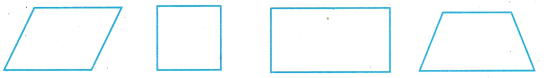The term ‘parallelogram’ is derived from Middle French ‘parallélogramme’, Late Latin ‘parallelogrammum’ and Greek ‘parallelogrammon’ which means “bounded by parallel lines”.Explanation:
In figures 1,2 and 3 opposite side are parallel to each other. so figure 1 , 2 and 3 are parallelograms in figure 4 the sides are not parallel, So figure 4 is not a parallelogram

Question 2.
You need to know the language of math in order to keep learning. In mathematics, you learn something new ¡n every grade. You learn something new in every module and lesson. In mathematics, you always build on what you know.

Think: I keep a log of all the math words I learn.

Writing: Preview Module 13. Make a list of words that are highlighted or in boldface type. Write “Yes” next to each word you know. Write “No” next to those that are new. Keep the list handy as you work on the lessons. Turn every “No” into a “Yes”!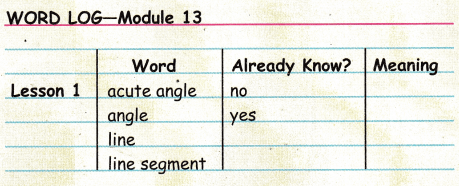What’s the Measure?

Object of the Game Compare angles to right angles to earn more points than the other player.

Materials

• Angle Cards

Set Up
Cut out the angle cards and place them face down in a stack.
Number of Players 2

How to play

Question 1.
Player 1 picks a card from the stack and decides if the angle shown is a right angle, is less than a right angle, or is greater than a right angle.

• If the angle is less than a right angle, Player 1 gets one point.
• If the angle is a right angle, Player 1 gets two points.
• If the angle is greater than a right angle, Player 1 gets three points.

Question 2.
Player 2 repeats Step 1. If a player incorrectly classifies an angle, the points go to the other player.